×#### Thank you for registering.

One of our academic counsellors will contact you within 1 working day.

Click to Chat

1800-1023-196

+91-120-4616500

CART 0

• 0

MY CART (5)

Use Coupon: CART20 and get 20% off on all online Study Material

ITEM
DETAILS
MRP
DISCOUNT
FINAL PRICE
Total Price: Rs.

There are no items in this cart.
Continue Shopping```Sequences

Meaning of Sequence

Sequence in Mathematics

Notation

Finite Sequence

Infinite Sequence

Rule of Sequence

Sequence with formula

Other Special Sequences

Arithmetic Sequence

Geometric Sequence

Fibonacci Sequence

Square Number Sequence

Cube Number Sequence

Triangular Numbers

Examples

Meaning of Sequence

A sequence is a collection of objects following some certain pattern.

ExampleHere in the above image it is the collection of shapes following a certain pattern, so that we can understand what could come next.

As in the first sequence the next object will be red triangle and in the second sequence the next object will be Yellow Square.

Sequence in Mathematics

A sequence is a list of numbers in an ordered form which follow a certain pattern. That order could be anything like backward, forward, alternate etc.The numbers in the list of sequence are called ‘term’ or ‘members’ or ‘elements’. It is like a set of numbers.

Notation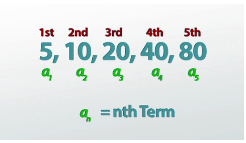The sequence can be referred by a capital letters like A or S.

Its terms could be denoted by an where n is the no. of terms. So that we can say that the first term of the sequence will be denoted by a1 and the second term will be denoted by a2 and so on. The nth term is the number at the nth place and it is shown by an. It is also called the General Term of the sequence. As in the above figure the terms are indicated by a1, a2, a3, a4 and a5.

Like set, it is also represented by listing all the elements in the required order, separated by commas and then embraces it in the curly brackets.

We can also write the sequence in form of its terms. For example, the sequence of terms ai, with the index moving from i = 1 to i = n, can be written as: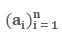The first value of the counter is called the "Lower Index"; the last value is called the "Upper Index".

Finite Sequence

Finite sequence is a sequence having limited or fixed number of terms.

Example

{1, 2, 3, 5, 7} is the sequence of first five prime numbers.

{m, a, r, y} is the sequence of letters in the name "mary".

Infinite Sequence

Infinite sequence is a sequence having unlimited number of terms or it has no end.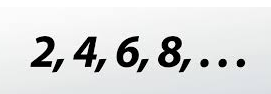Example

This is the infinite sequence of even numbers. Here the three dots show that it goes on forever. So its last term will be represented by n∞.

In the other form it can be written as: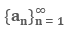Here ∞ the infinity symbol shows that this is an infinite sequence.

Rule of Sequence

Generally all the sequences follow some rule which helps us to find the value of all the terms of the sequence using some algebraic formula.

Example

Let’s see the example of sequence of even natural numbers.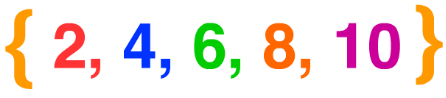Here it is a sequence of even natural numbers till 10.As we can see it is following a certain pattern.

a1  = 2

2 × 1

a1  = 4

2 × 2

a1  = 6

2 × 3

a1  = 8

2 × 4

a1  = 10

2 × 5

Here we can see that we can write the nth term as 2n where n is a natural number.

Hence the formula for calculating the value of any nth term for the sequence of even natural numbers would be very easy by the formula

an = 2n

Sequence with formula

As we know that every sequence follows some rule, so we must have a formula for it. Hence if we know the formula of nth term then we can easily build the sequence.

Example

Let's build the sequence whose nth term is given by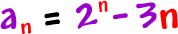Let n = 1, to get the first term of the sequence:

a1 = 21 -3(1) = -1

Let n = 2, to get the second term:

a2 = 22 -3(2) = -2

Let n = 3, to get the third term:

a3 = 23 -3(3) = -1

and so on...

So, our sequence is

-1,-2,-1, 4, 17, 46,…

Other Special Sequences

Arithmetic Sequence

An arithmetic sequence is that sequence in which the difference between two successive terms is always constant. It could be by adding or subtracting a constant number. But it should be by adding or subtracting only.

Example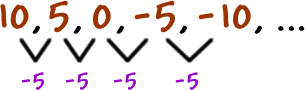Here in this infinite sequence we can see that we are getting the next terms by subtracting 5. As the difference is same that is, -5 in the whole sequence, so this is an arithmetic sequence.

Example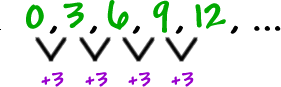Here we are getting the terms by adding 3 every time .This number 3 is called the difference because this is the difference between the two successive terms.

The difference is denoted by “d”.

Like here we can see that a1 =0 and a2 = 3.

The difference between the two is

a2 – a1 =3

a3 – a2 =3

If the first term of an arithmetic sequence is a1 and the common difference is d, then the nth term of the sequence is given by:

an= a1+ (n−1) d

Geometric Sequence

As in arithmetic sequence we add or subtract to get the next term but in geometric sequence we multiply with a same number every time to get the next term of the sequence.

Example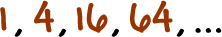Here

a1 =1

a2 = 4 = a1(4)

a3 = 16 = a2(4)

In this sequence we multiply it with 4 every time to get the next term. Here the number 4 is called the Ratio.

The ratio is denoted by “r”.

an = an−1⋅r or an = a1⋅rn−1

Fibonacci Sequence

In the Fibonacci sequence there is no visible pattern but its successive terms comes by adding the value of the two terms before the required term.

Example

0, 1, 1, 2, 3, 5, 8, 13, 21, 34, ...

In the above sequence we can see

a1 = 0 and a2 = 1

a3 = a2 + a1 = 0 + 1 = 1

a4 = a3 + a2  = 1 + 1 = 2 and so on.

So the formula of the Fibonacci sequence is

an = an – 2 + an – 1, n > 2

This is also called the Recursive Formula.

Square Number Sequence

Square numbers are the squares of the whole numbers:

0, 1, 4, 9, 16, 25, 36, 49, 64, 81, …

0 ( = 0 × 0)
1 ( = 1 × 1)
4 ( = 2 × 2)
9 ( = 3 × 3)
16 ( = 4 × 4)
etc...

Cube Number Sequence

Cube Numbers are the cubes of the whole numbers starting from 1.

1, 8, 27, 64, 125, 216, 343, 512, 729, …

1 ( = 1 × 1 × 1)
8 ( = 2 × 2 × 2)
27 ( = 3 × 3 × 3)
64 ( = 4 × 4 × 4)
etc...

Triangular Numbers

The Triangular Number Sequence is created from a pattern of dots which form a triangle.

1, 3, 6, 10, 15, 21, 28, 36, 45, …

By adding another row of dots and counting all the dots we can find the next number of the sequence: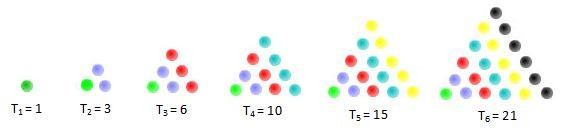Examples

Example 1: Find the common difference and the next term of the following sequence:

2, 7, 12, 17, 22,…

Solution: To find the common difference we have to subtract the successive numbers

7 – 2 = 5

12 – 7 = 5

17 – 12 = 5

22 – 17 = 5

Here the difference is always 5 so the common difference is d = 5

To find the next term we have to add the common difference to the fifth term.

22 + 5 = 27

So the next term is 27.

Example 2: Find the first four terms of a geometric sequence in which a1 = 3 and r = 4.

Solution: Given

a1 = 3 and r = 4

formula of the geometric sequence is

an = an−1⋅r

a1 =3

a2 = 3 × 4 = 12

a3 = 12 × 4 = 48

a4 = 48 × 4 = 192

Example 3: Find the next term of the given Fibonacci sequence.

0, 1, 1, 2, 3, 5, 8, 13, 21, 34, ...

Solution: To find the next term that is, n11, we will use the formula.

an = an – 2 + an – 1

a11 = a10+ a9

= 34 + 21

= 55

Example 4: Find the tenth term and the n-th term of the following sequence:

1, 2, 4, 8,…

Solution: To find the next term i.e. n11, we will use the formula.

an = an – 2 + an – 1

a11 = a10+ a9

= 34 + 21

= 55

Example 5: Find the tenth term and the n-th term of the following sequence:

1, 2, 4, 8,…

Solution: First of all we have to check whether it is arithmetic or geometric sequence.

We can see that the differences of two successive terms are not constant.

2 – 1 = 1z

4 – 2 = 2

8 – 4 = 4

So it’s not an arithmetic sequence.

Now let’s check the ratio of the successive terms.

2/1 = 2

4/2 = 2

8/4 = 2

Where, common ratio r = 2, and the first term is a = 1

To find the n-th term, we will use the  formula an = ar(n – 1)

an = ar(n – 1)

an = 1.2(n – 1)

an = 2 (n – 1)

To find the value of the tenth term, we will put the value n = 10 into the n-th term formula:

a10 = 2(10 – 1)

= 29

= 512

So it is a geometric sequence.

Sequences
```### Course Features

• 731 Video Lectures
• Revision Notes
• Previous Year Papers
• Mind Map
• Study Planner
• NCERT Solutions
• Discussion Forum
• Test paper with Video Solution